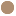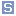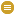ObjectPropertyArgument used to introduce numbers into the unit of measure description where they are needed (e.g., 4 where the measure needs an argument such as CEMI(n=4)). Most arguments used in practice however will be integers (i.e., 'denominator'=1). Value 0 in 'numerator' and 'denominator' means not applicable.cim:ReadingTypecim:RationalNumber